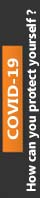Sep 25, 2017 term paper 2

# POD MODELS PARTIAL ONE-DIMENSIONAL MEAN FUNCTIONS FOR PROBLEMS WITH BOTH FACTORS AND CONTINUOUS…

This paper concentrates on the primary theme of POD MODELS PARTIAL ONE-DIMENSIONAL MEAN FUNCTIONS FOR PROBLEMS WITH BOTH FACTORS AND CONTINUOUS… in which you have to explain and evaluate its intricate aspects in detail. In addition to this, this paper has been reviewed and purchased by most of the students hence; it has been rated 4.8 points on the scale of 5 points. Besides, the price of this paper starts from £ 40. For more details and full access to the paper, please refer to the site.

POD models Partial one-dimensional mean functions for problems with both factors and continuous predictors were discussed in Section 6.4. For the Australian athletes data discussed in that section, the mean function (6.26),was suggested. This mean function is nonlinear because η1 multiplies each of the βs. Problem 6.21 provides a simple algorithm for finding estimates using only standard linear regression software. This method, however, will not produce the large-sample estimated covariance matrix that is available using nonlinear least squares.

Problem 6.21

A POD model for a problem with p predictors X = (X1 …,Xp) and a factor F with d levels is specified, for the jth level of F, byThis is a nonlinear model because ηij multiplies the parameter β. Estimation of parameters can use the following two-step algorithm:

1. Assume that the η1j , j = 1,…,d are known. At the first step of the algorithm, set η1j = 1, j = 1,…,d. Define a new term zj = η1j x, and substituting into (6.30),We recognize this as a mean function for parallel regressions with common slopes β and a separate intercept for each level of F. This mean function can be fit using standard ols linear regression software. Save the estimate2. Let v =(6.30) to getwhich we recognize as a mean function with a separate intercept and slope for each level of F. This mean function can also be fit using ols linear regression software. Save the estimates of η1j and use them in the next iteration of step 1.

Repeat this algorithm until the residual sum of squares obtained at the two steps is essentially the same. The estimates obtained at the last step will be the ols estimates for the original mean function, and the residual sum of squares will be the residual sum of squares that would be obtained by fitting using nonlinear least squares. Estimated standard errors of the coefficients will be too small, so t-tests should not be used, but F-tests can be used to compare models.

Write a computer program that implements this algorithm.

1.       Describe a reasonable method for finding starting values for fitting (6.26) using nonlinear least squares.2.       For the cloud seeding data, Problem 9.11, fit the partial onedimensional model using the action variable A as the grouping variable, and summarize your results.

Problem 9.11

The data in the file cloud.txt summarize the results of the first Florida Area Cumulus Experiment, or FACE-1, designed to study the effectiveness of cloud seeding to increase rainfall in a target area (Woodley, Simpson, Biondini, and Berkeley, 1977). A fixed target area of approximately 3000 square miles was established to the north and east of Coral Gables, Florida. During the summer of 1975, each day was judged on its suitability for seeding. The decision to use a particular day in the experiment was based primarily on a suitability criterion S depending on a mathematical model for rainfall. Days with S > 1.5 were chosen as experimental days; there were 24 days chosen in 1975. On each day, the decision to seed was made by flipping a coin; as it turned out, 12 days were seeded, 12 unseeded. On seeded days, silver iodide was injected into the clouds from small aircraft. The predictors and the response are defined in Table 9.3.

The goal of the analysis is to decide if there is evidence that cloud seeding is effective in increasing rainfall. Begin your analysis by drawing appropriate graphs. Obtain appropriate transformations of predictors. Fit appropriate mean functions and summarize your results. (Hint: Be sure to check for influential observations and outliers.)0% Plagiarism Guaranteed & Custom Written, Tailored to your instructions

International House, 12 Constance Street, London, United Kingdom,
E16 2DQ

## STILL NOT CONVINCED?

We've produced some samples of what you can expect from our Academic Writing Service - these are created by our writers to show you the kind of high-quality work you'll receive. Take a look for yourself!FLAT 25% OFF ON EVERY ORDER.Use "FLAT25" as your promo code during checkout